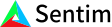1. Bob Anderson
×
IntuitiveML

## Terms: Nonlinearity

Jun 4, 2020

When learning about neural networks for the first time, you might hear about the term nonlinearity around the time you learn about activation functions. Basically, non-linearity just means not linear.

While nonlinear does mean not linear, there are a couple of small catches that aren’t obvious right away. For example, if the output function of a network could be described by the function sqrt(2)*x^2+ pi^3sin(x) you might think that the function is doing a nonlinear transformation. However, if the inputs into the network are x^2 and sin(x), then you know that the network did a linear transformation. It’s a linear transformation since the output can be written as a linear combination of the inputs – that is, if we say y = x^2 and z = sin(x), we can immediately see that sqrt(2)*y + pi^3*z is a linear function of y and z. Note that we don’t care that the constants are the sqrt(2) and pi^3, those are just constants that don’t depend on the input – we can rewrite this function as f(y,z)= ay+bz and see more clearly that this is a linear function. You might now be confused – so if the output can be nonlinear but it isn’t a nonlinear transformation, then what is a nonlinear transformation?

Anything that can’t be written as the sum of all the inputs times some constants. That is, f(x,x2,x3…) is linear only if it can be written as f(x,x2,x3…) = a1x1 + a2x2 + a3x3… + c (some constant c).

Going back to the earlier example, that example is only linear if the inputs are x^2 and sin(x). If the input is x by itself it’s nonlinear, x^2 by itself it’s nonlinear, or sin(x) by itself it’s nonlinear, we know it’s a not a linear transformation because there is no way to take x^2 and multiply it or add some constant to it to get sin(x) or vice versa.